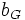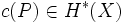# Characteristic class

Let$G$ be a topological group. A characteristic class of principal$G$-bundles is a natural transformation from the contravariant functor$b_G$ (which sends any topological space to the set of isomorphism classes of principal$G$-bundles on it) to the cohomology functor.
For a given topological space$X$, a characteristic class of principal$G$-bundles associates, to every principal$G$-bundle$P \to X$, an element$c(P) \in H^*(X)$, such that if$f:X \to Y$ is a continuous map, then$c(b_g^*(f)(P)) = H^*(f)(c(P))$.